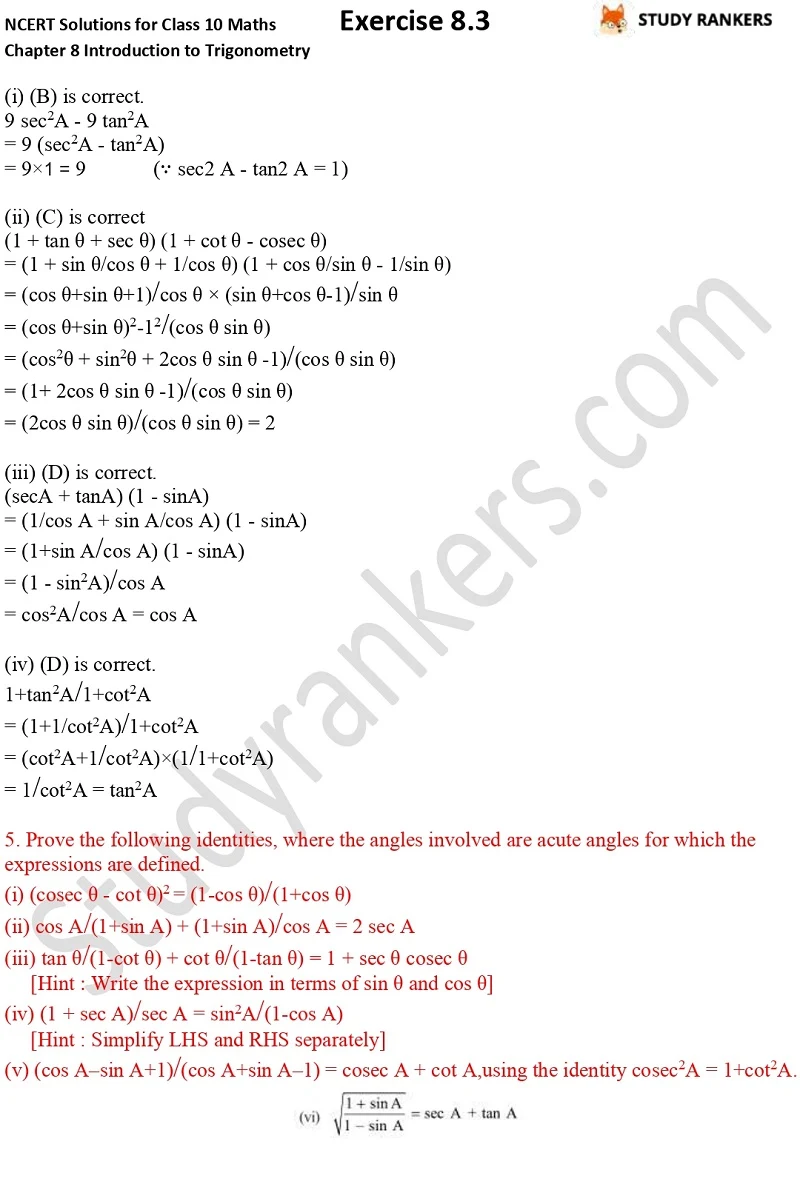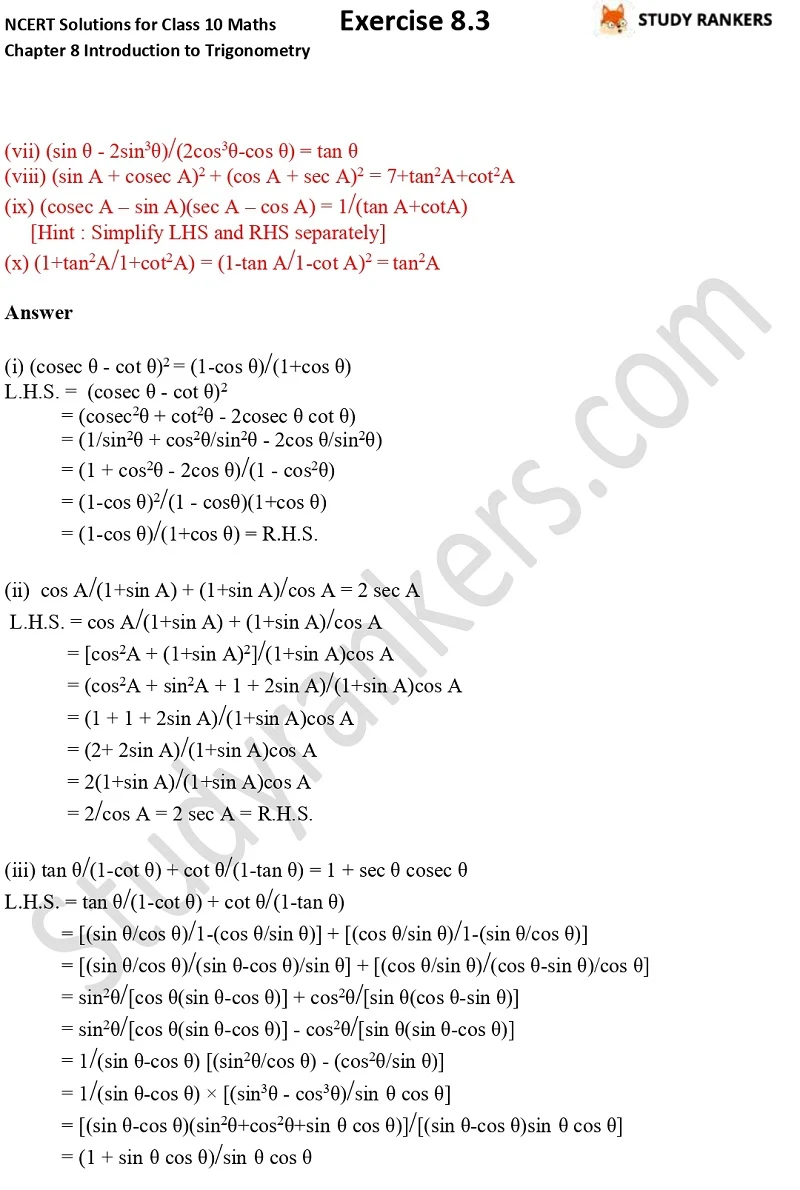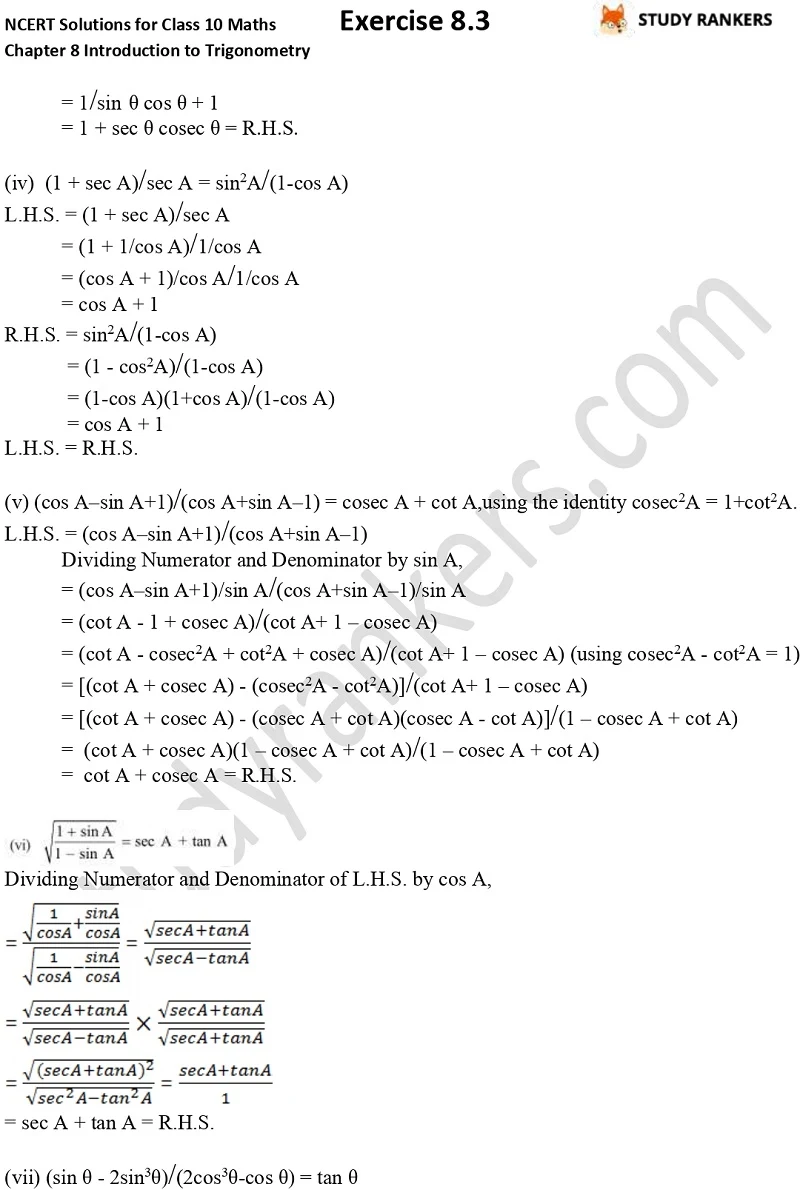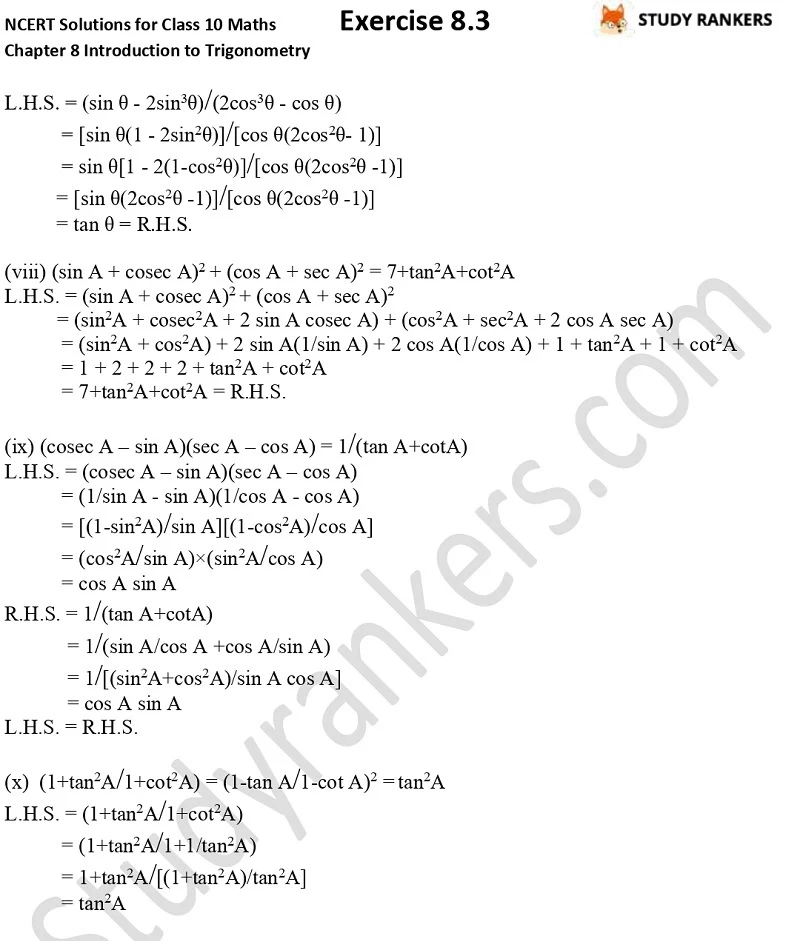## NCERT Solutions for Class 10 Maths Chapter 8 Introduction To Trigonometry Exercise 8.4

Class 10 Maths NCERT Solutions for Chapter 8 Introduction To Trigonometry Exercise 8.4 provided here is very essential for class 10 students that will helpful in understanding the basic terms and formula to solve problems. It will improve problem solving skills of students as well as boost their confidence level. NCERT Revision Notes for Class 10 will also help in revising the important points of chapter before examinations. These Maths Class 10 Solutions will prove very useful in completing your homework at time also.

Exercise 8.4 contains total five questions in which you have to prove the given identities, where the angles involved are acute angles for which the expressions are defined.